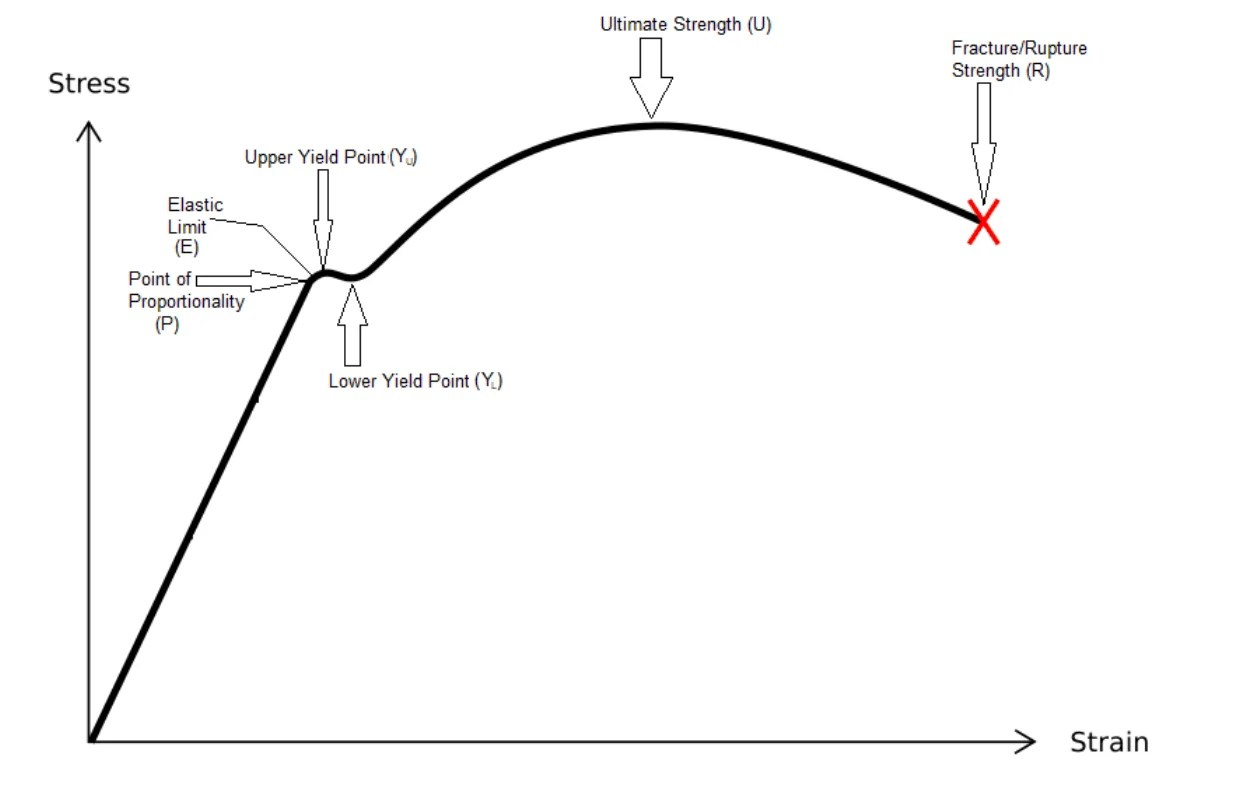# Tensile Test or Tensile Testing [Explained with Graph]

Today, in this post, I am going to discuss a very important topic of material testing. That important topic is the Tensile test. Below I have explained it in detail. So just keep reading…

## Tensile Test

Tensile Test is a mechanical test in which a tensile force is applied at both of the ends of a specimen of the material. This test is performed to calculate or measure the tensile strength and other tensile properties of a material. It is also called Tension Test or Tension Testing.

Usually, this test is performed on two types of material. These types are as follows:

1. A material with yield point phenomenon (An example is plain carbon steel)
2. A material without yield point phenomenon (An example is an aluminum alloy)

## Machine Used for Tensile Testing

This test is executed in a material testing laboratory. Generally, a testing machine named Universal Testing Machine (abbreviated as UTM) is used for performing the tensile test.

As shown in the image displayed above, The UTM has two crossheads. The specimen is gripped in between these two crossheads. A device named as Extensometer is there in a UTM. This device performs the task of measuring the elongation or strain in the specimen.

## Stress-Strain Diagram

When we perform a tensile test, a graph or diagram is plotted. This graph shows the relationship between the force applied and the elongation observed in the material during the test. Or you can say that it shows a relationship between the stress and strain developed in the material during the test.As shown in the image given above, from this Tensile Test Graph, you can also identify the Yield Strength, Ultimate Tensile Strength, Break or Rupture Point, Point of proportionality, Elastic Limit of the material, etc. All of these terms are explained below:

Point of Proportionality(P): The proportionality point is the point up to which the stress is directly proportional to strain during the testing process.

Elastic Limit(E): The point up to which the material behaves as an elastic material. Up to this stage, if the load is removed, the specimen can regain its original shape and size.

Yield Point (Y): The point at which the plastic deformation starts taking place in the material during the test is called the Yield Point (P). The value of the stress developed at this point is called the Yield Strength of the material. Different materials have a different value for the Yield Strength.

Ultimate Tensile Strength/Ultimate Strength(U): The maximum amount of force that a material can withstand (before fracture) per unit cross-sectional area is called the Ultimate Strength of the material. You can also say that it is the maximum tensile stress developed in the material during the tensile testing. The value of the Ultimate Strength is different for different material.

Fracture/Rupture Strength(R): The point at which the material breaks or fracture during the testing process is called the Rupture Point. And, the amount of stress at that point is called the Rupture Strength of the material.

## Specimen

A specimen is actually a sample piece of the material which you want to test. For Tensile Testing, generally, a specimen with two threaded shoulders is used. The diameters of the shoulders are higher than the diameter of the portion of the specimen between the two shoulders.The portion between the shoulder also contains the gauge portion or section. Guage Portion is the portion of the specimen which undergoes elongation during the test. The length of the gauge portion is called the Guage Length.

## Testing Process

In the testing process, the specimen to be tested is held in between the two crossheads of the machine. The grips in the machine clamp the specimen. The load is applied and increased gradually. The specimen elongates while the testing process continues. It continues until the specimen breaks. The relationship between the elongation in the gauge portion with respect to the force applied is recorded. This data helps the machine to plot the Stress-Stress Diagram.

I hope you read this article with full attention and everything is clear now. But still, if you need any help about the topic, use our comment section below. All of your queries will surely be answered.

Also, go through the following articles also in order to enhance your knowledge in this field:

Tensile Test of Mild Steel

Tensile Strength Meaning, Definition, Symbol, Formula and Unit

### 2 thoughts on “Tensile Test or Tensile Testing [Explained with Graph]”

1.2.shubhangi srivastava

HELLO SIR,
thankyou for explaining well but I want to know that how this graph is plotted in tensile tester software, may you please explain me about the tensile tester software horizon.